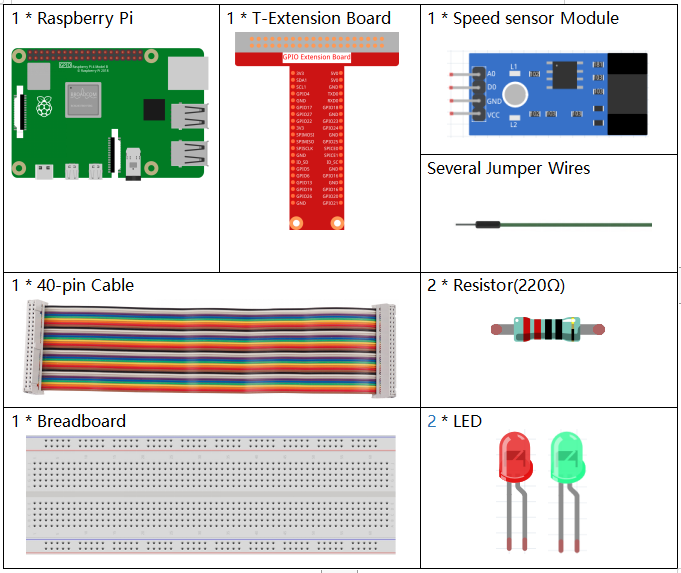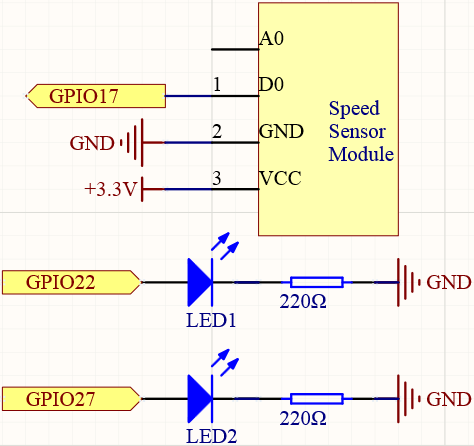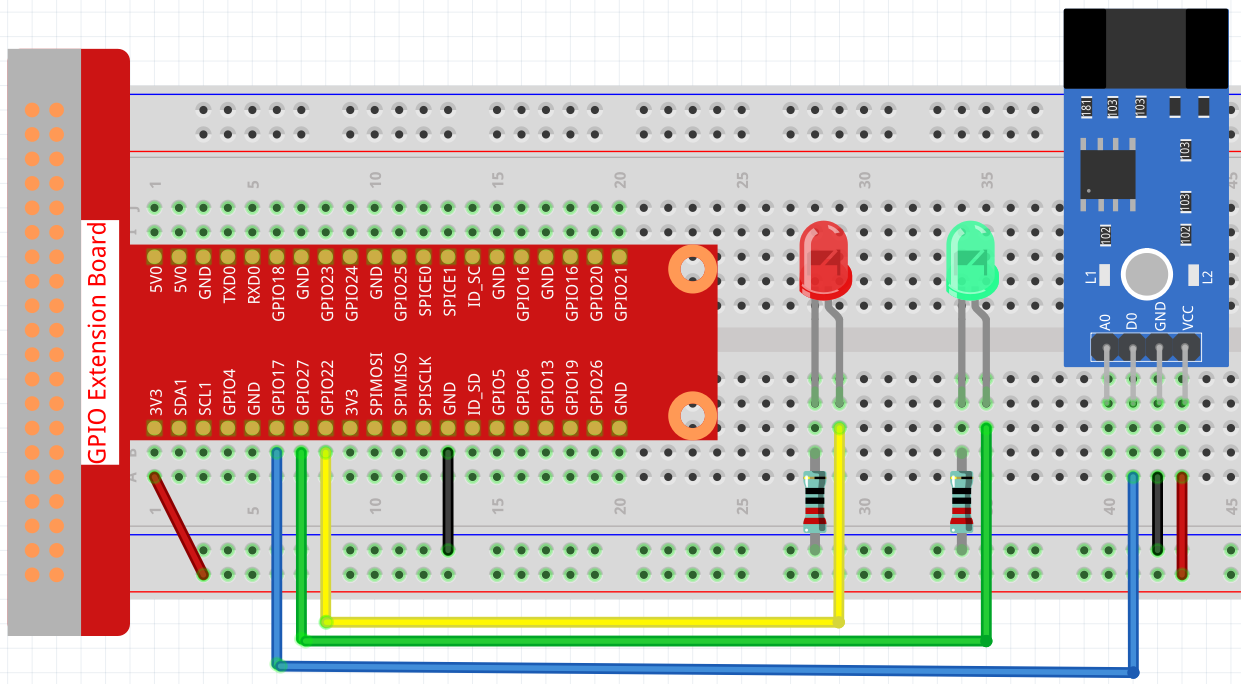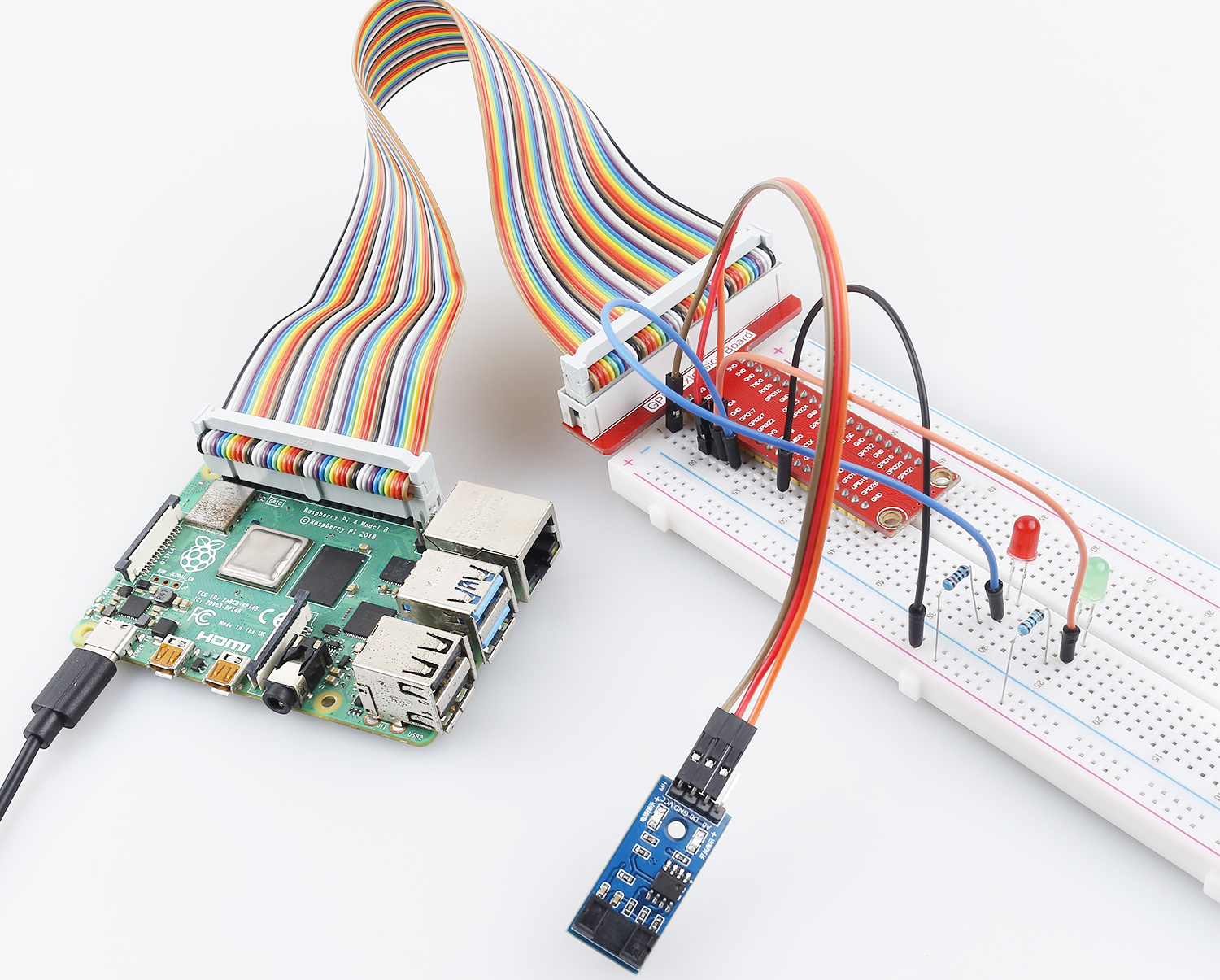# 2.2.6 Speed Sensor Module¶

## Introduction¶

In this project, we will learn the use of the speed sensor module. A Speed sensor module is a type of tachometer that is used to measure the speed of a rotating object like a motor.

## Required Components¶

In this project, we need the following components.Name

ITEMS IN THIS KIT

Raphael Kit

337

Raphael Kit

COMPONENT INTRODUCTION

GPIO Extension Board

Jumper Wires

Resistor

LED

Speed Sensor Module

-

## Schematic Diagram¶## Experimental Procedures¶

Step 1: Build the circuit.Step 2: Change directory.

```cd ~/raphael-kit/python
```

Step 3: Run.

```sudo python3 2.2.6_speed_sensor_module.py
```

After the code runs, the green LED will light up. If you place an obstacle in the gap of the speed sensor module, the “light blocked” will be printed on the screen and the red LED will be lit. Remove the obstacle and the green LED will light up again.

Code

Note

You can Modify/Reset/Copy/Run/Stop the code below. But before that, you need to go to source code path like `raphael-kit/python`. After modifying the code, you can run it directly to see the effect.

```#!/usr/bin/env python3
import RPi.GPIO as GPIO

speedPin  = 17
Gpin   = 27
Rpin   = 22

def setup():
GPIO.setmode(GPIO.BCM)       #
GPIO.setup(Gpin, GPIO.OUT)     # Set Green Led Pin mode to output
GPIO.setup(Rpin, GPIO.OUT)     # Set Red Led Pin mode to output
GPIO.setup(speedPin, GPIO.IN, pull_up_down=GPIO.PUD_UP)    # Set speedPin's mode is input, and pull up to high level(3.3V)

def Led(x):
if x == 0:
GPIO.output(Rpin, 0)
GPIO.output(Gpin, 1)
if x == 1:
GPIO.output(Rpin, 1)
GPIO.output(Gpin, 0)
print ('Light was blocked')

def detect(chn):
Led(GPIO.input(speedPin))

def loop():
while True:
pass

def destroy():
GPIO.output(Gpin, GPIO.LOW)       # Green led off
GPIO.output(Rpin, GPIO.LOW)       # Red led off
GPIO.cleanup()                     # Release resource

if __name__ == '__main__':     # Program start from here
setup()
try:
loop()
except KeyboardInterrupt:  # When 'Ctrl+C' is pressed, the child program destroy() will be  executed.
destroy()
```

Code Explanation

```GPIO.add_event_detect(speedPin, GPIO.BOTH, callback=detect, bouncetime=200)
```

Add an event here, triggered by a change in the level of `speedPin` and call `detect()` to control the 2 LEDs on and off.

```def Led(x):
if x == 0:
GPIO.output(Rpin, 0)
GPIO.output(Gpin, 1)
if x == 1:
GPIO.output(Rpin, 1)
GPIO.output(Gpin, 0)
print ('Light was blocked')
```

Define a function `Led()` that turns the red LED on and prints `Light was blocked` when the parameter is 1; turn the green LED on when the parameter is 0.

```def detect(chn):
Led(GPIO.input(speedPin))
```

Define a callback function where the value of `speedPin` will control the turning on or off of the 2 LEDs.

## Phenomenon Picture¶